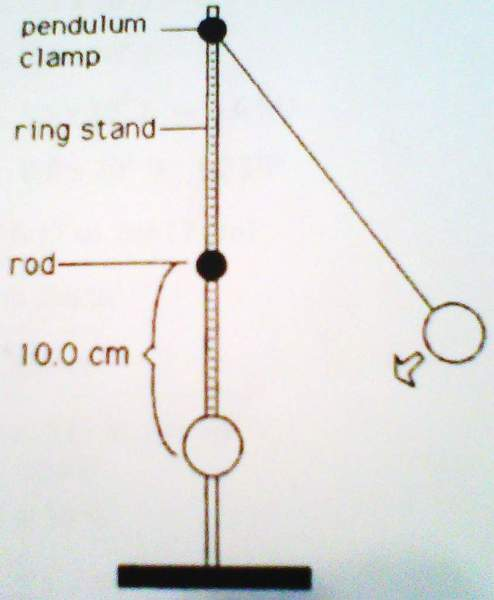# Pendulum bob must circle rodThe pendulum bob in the above figure must circle the rod interrupting its swing, and the string must remain taut at the top of the swing. How far up must the bob be raised before releasing it to accomplish these goals?

I don't know where to begin with this because I don't quite understand what i'm supposed to find.. I've tried finding Ep and Ek using 1/2mv2=mgh but i don't seem to have enough info.

Thank you in advance for any suggestions.

rock.freak667
Homework Helper
Well from ½ mv2 = mgh, what would v2 be equal to?

Since the distance of the rod to the bottom of the swing is 10 cm, what is the centripetal force required? (in term of 'm')

At the top of the swing what are the forces acting and in what directions are they acting? (The resultant of these two will be the same as the centripetal force required)

Well from ½ mv2 = mgh, what would v2 be equal to?

Since the distance of the rod to the bottom of the swing is 10 cm, what is the centripetal force required? (in term of 'm')

At the top of the swing what are the forces acting and in what directions are they acting? (The resultant of these two will be the same as the centripetal force required)

the velocity is equal to 1.4m/s and the force acting upon the bob is gravity... but don't i need to know the mass of the bob in order to figure out the force of gravity? still not sure where to go with it..

rock.freak667
Homework Helper
the velocity is equal to 1.4m/s and the force acting upon the bob is gravity... but don't i need to know the mass of the bob in order to figure out the force of gravity? still not sure where to go with it..

At the top you will have both tension and gravity acting the same direction.

So T + mg = mv2/r or T = mv2/r - mg.

For the string to be taut T≥ 0.

Also in v2 = 2gh, 'h' is not necessarily 10 cm since that would mean you've already found 'h' when you're in fact trying to find 'h'.

I'm really not understanding this at all, thanks though! I guess i'll just leave it.